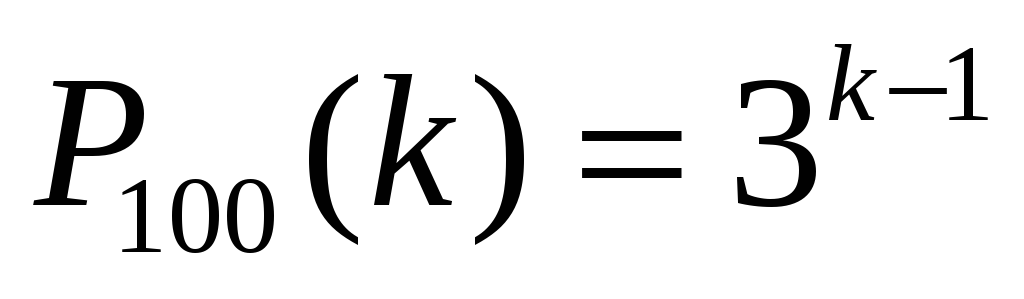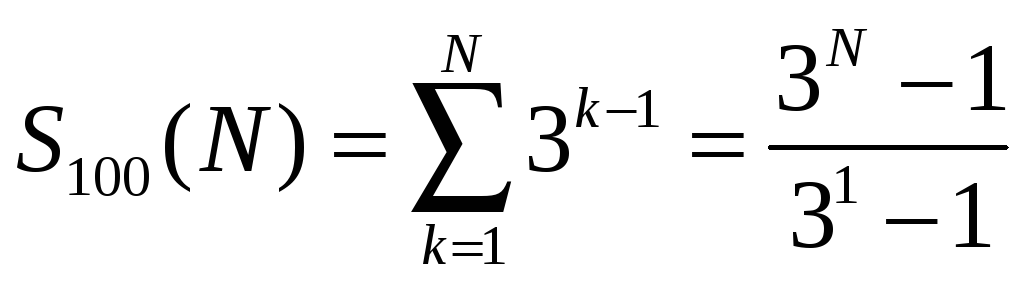# Table 3: Explicit description of the moves to solve the N=2 CMToH

 Page 6/20 Date 23.04.2018 Size 2.78 Mb. #45758

Table 3: Explicit description of the moves to solve the N=2 CMToH puzzle. The total number of moves for both versions is 4. And the N=3 case of the CMToH puzzle is solved by 13 moves.

1. Expressions for the number of moves

Simple observations reveal that, as is the case with the classical ToH, the "forward" moves solving the CMToH puzzle are deterministic.

Furthermore, it is not too difficult to show by a recursive argument (see the proof for the classical ToH[2,3]) that the number of disk moves P100(k) and (therefore) the total number of moves P100(N) perfectly span base 3:. (7)
and. (8)
The subscript "100" in Equations 7 and 8, relates to a solution "duration" of 100%.

Table 4 lists the (minimum) number of moves of each disk (Equation 7) and the total (minimum) number of moves (Equation 8) required to solve the CMToH puzzle for the first eight stack heights.

 k N 1 2 3 4 5 6 7 8 SUM (3N - 1)/2 1 1 1 1 2 1 3 4 4 3 1 3 9 13 13 4 1 3 9 27 40 40 5 1 3 9 27 81 121 121 6 1 3 9 27 81 243 364 364 7 1 3 9 27 81 243 729 1093 1093 8 1 3 9 27 81 243 729 2187 3280 3280